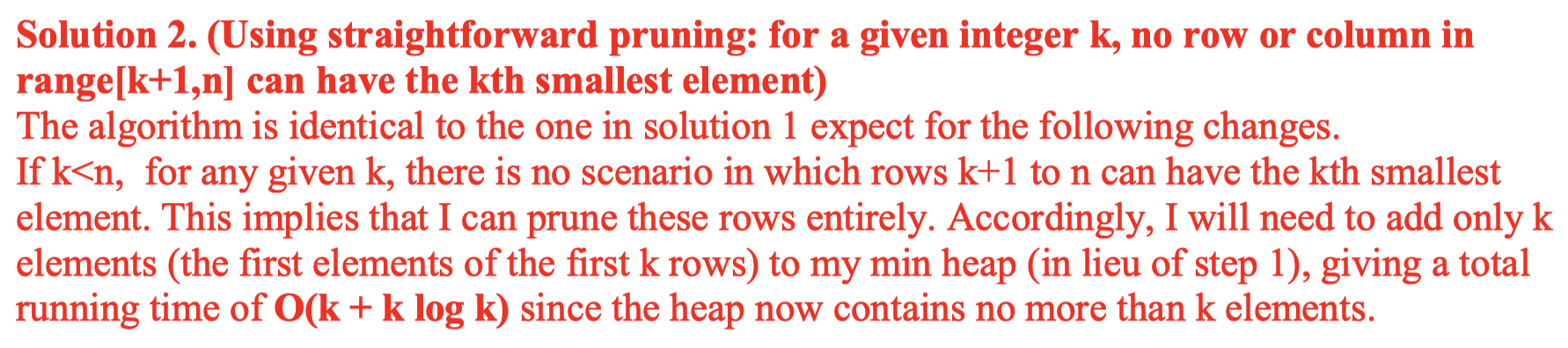# 378. Kth Smallest Element in a Sorted Matrix

Reference: LeetCode EPI 11.6
Difficulty: Medium

## Problem

Given a n x n matrix where each of the rows and columns are sorted in ascending order, find the kth smallest element in the matrix.

Note that it is the kth smallest element in the sorted order, not the kth distinct element.

Note: You may assume $k$ is always valid, $1 ≤ k ≤ n^2$.

Example:

## Analysis

### Brute-Force

Time: $O(n^2\log{k})$
Space: $O(k)$

### Row Heap

• Add the first row into the priority queue. Poll values from it for k times then the last one we poll will be the kth smallest.
• Each time we poll a value from it, we should offer its next value if available.

Note: We can also use the column.

Time: $O(n\log{k})$
Space: $O(k)$

If we use bottom-up heapification, it should be $O(n + k\log{n})$

If $k < n$, we can have pruning:Reference:

The key point for any binary search is to figure out search space. There are two kinds of search space: index and range. Usually, when the array is sorted in one direction, we can use index as search space; otherwise, we use values as our search space.

In this case, we cannot use index as our search space, because the matrix is sorted in two directions, we can not find a linear way to map the number and its index.

Time: $O(n\log{n}\log{N})$

• $N$ is the search space that ranges from the smallest to the largest element.
• Each count takes $O(n\log{n})$ in the best case when mid is getting smaller.
• Each count takes $O(n^2)$ in the worst case when mid is getting larger.

Space: $O(1)$

Improvement:

We can actually improve count function.

Time: $O(n\log{N})$
Space: $O(1)$

Count by row starting from up-right:

Count by column starting from bottom-left:

CommentJunhao Wang
a software engineering cat